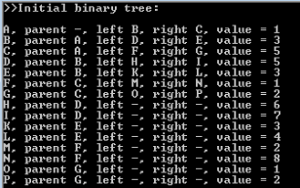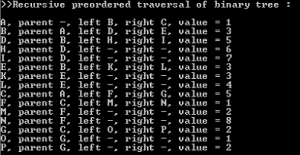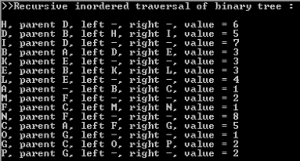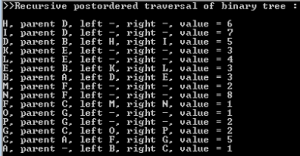# Example of Alpha Beta Pruning: Part 2

Create a binary tree of 15 nodes which use the following names:

```               [A]
/        \
[B]             [C]
/     \         /    \
[D]    [E]      [F]    [G]
/  \   /   \    /  \   /  \
[H] [I] [J] [K] [L] [M] [N] [O]
```

with the nodes holding the following values:

```               
/        \
             
/     \        /      \
              
/  \   /   \   /   \   /   \
       
```
• The binary tree must be created automatically from an array containing the values: {1,3,5,5,3,1,2,6,7,3,4,2,8,1,2}.
• Display the tree in console window showing the following information: node name, parent node name, children node names, and the value stored in each node. Hyphenate the non-existing parent/children nodes.
• Perform a preordered traversal of binary tree, and display the order nodes were traversed.
• Perform an inordered traversal of binary tree, and display the order nodes were traversed.
• -Perform a postordered traversal of binary tree, and display the order nodes were traversed.

SOLUTION:

For the sake of reference, we are going to use the same tree as in the previous sample, in order to show the different ways to traverse the tree. First of all, we will need to create a tree structure consisting from nodes. Each node can have a parent node, and (since its Binary tree) two children nodes: left and right. Each node also has a name, and a value it carries. Node class will look as following:

```public class Node
{
public Node left { get; set; }
public Node right { get; set; }
public Node parent { get; set; }
public string name { get; set; }
public int value { get; set; }

public Node(Node p, string n, int v)
{
parent = p;
name = n;
value = v;
}
}
```

The pre-requisites for creation of our binary tree include a pair of arrays (one housing values and the other names of nodes):

```//Array of binary tree node values:
int[] arr = new int[] { 1, 3, 5, 5, 3, 1, 2, 6, 7, 3, 4, 2, 8, 1, 2 };
//Array of letter markers for discerning the nodes easier:
string[] arr2 = new string[] { "A", "B","C", "D","E","F","G", "H","I","K","L","M","N","O","P" };
```

Some other pre-requisites:

```//Array which will house the number of nodes required:
Node[] tree = new Node[arr.Length];
//Nonexisting parent of the very first node in the tree:
Node parent = null; //We will use this variable to store the parents of subsequent nodes as well
```

We will go through the “tree” array, creating the nodes. To create the node, we need to provide a parent node, name, and numerical value respectively:

```tree[i] = new Node(parent, arr2[i], arr[i]);
```

But we should take into account that the “null” parent is only fitting for the very first “parentless” node “A”. For all subsequent nodes, we need a mechanism that will assign proper parents to the next branches of 2, 4, 8 nodes and so on:

```if (i > 0) parent = tree[(i + (i % 2 == 0 ? 0 : 1) - 2) / 2];
```

Which makes for the following array-filling code:

```for (int i = 0; i < arr.Length; i++)
{
if (i > 0) parent = tree[(i + (i % 2 == 0 ? 0 : 1) - 2) / 2];
tree[i] = new Node(parent, arr2[i], arr[i]);
}
```

We are using arr.Length and not tree.Length since all of the arrays in this application have same length anyway. At this moment, we should have 15 nodes with “parent” attribute being filled. However, we have no children nodes yet. To fix this, we need to iterate through this array once again:

```//setting children nodes now that the every required node was created
for (int i = 0; i < arr.Length; i++)
{
//the last half of nodes lacks further children nodes, so they are set to null
tree[i].left = (i < arr.Length / 2) ? tree[i + i + 1] : null;
tree[i].right = (i < arr.Length / 2) ? tree[i + i + 2] : null;
} ```

Now we need to display the binary tree (which we will do on node-per-line basis), and for this we will create the appropriate function:

```static void ShowNodeData(Node n)
{
Console.Write("{0}, ", n.name);
if (n.parent != null) Console.Write("parent {0}, ", n.parent.name);
else Console.Write("parent -, ");
if (n.left != null) Console.Write("left {0}, ", n.left.name);
else Console.Write("left -, ");
if (n.right != null) Console.Write("right {0}, ", n.right.name);
else Console.Write("right -, ");
Console.WriteLine("value = {0}", n.value);
}
```

Calling this function from main part of application for every node in our array allows to display data about the whole binary tree:

```//displaying the binary tree
Console.WriteLine(">>Initial binary tree:");
Console.WriteLine();
foreach (Node item in tree)
{
ShowNodeData(item);
}
```

Result:Now we can move on performing the various types of traversal of our binary tree. The three types of traversal we are going to attempt are known as:

Preorder: Visit the root first, traverse the left subtree, then traverse the right subtree

Inorder: Traverse the left subtree first, then visit the root, then traverse the right subtree

Postorder: Traverse the left subtree first, then traverse the right subtree, then visit the root

The next function performs the preordered traversal of tree:

```static void recPreOrder(Node n)
{
//preordered traversal of binary tree - recursive
ShowNodeData(n);
if (n.left != null) recPreOrder(n.left);
if (n.right != null) recPreOrder(n.right);
}
```

Calling this function:

```Console.WriteLine(">>Recursive preordered traversal of binary tree :");
Console.WriteLine();
recPreOrder(tree);
```

results in:The next function performs the inordered traversal of tree:

```static void recInOrder(Node n)
{
//inordered traversal of binary tree - recursive
if (n.left != null) recInOrder(n.left);
ShowNodeData(n);
if (n.right != null) recInOrder(n.right);
}
```

Calling this function:

```Console.WriteLine(">>Recursive inordered traversal of binary tree :");
Console.WriteLine();
recInOrder(tree);
```

results in:The next function performs the postordered traversal of tree:

```static void recPreOrder(Node n)
{
//postordered traversal of binary tree - recursive
if (n.left != null) recPostOrder(n.left);
if (n.right != null) recPostOrder(n.right);
ShowNodeData(n);
}
```

Calling this function:

```Console.WriteLine(">>Recursive postordered traversal of binary tree :");
Console.WriteLine();
recPostOrder(tree);
```

results in:Therefore, we have displayed all three types of binary tree traversals, as was required.

This is the second part of an example of alpha beta pruning algorithm created by our programming specialists. We remind you that any usage of this information without proper acknowledgments is prohibited. But we won’t leave you alone to suffer with your programming assignment! Place your order and receive your personal assignment done by our professionals according to all your demands. We work not only with programming, but with a wide range of school, college and university disciplines. We can solve the most complex problems for you! To see the third part of our example of alpha beta pruning click here.